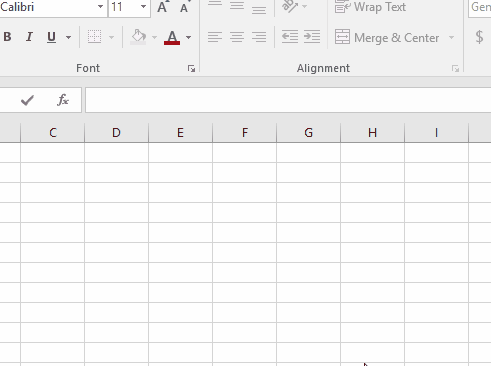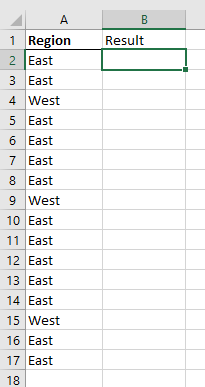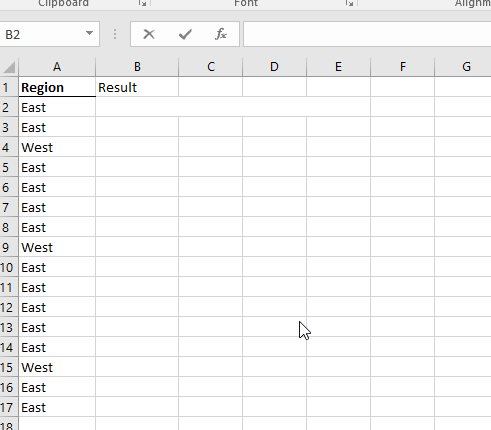# How to use NOT function in Microsoft Excel

The NOT function returns the opposite boolean value. NOT function in excel returns TRUE if False and False if TRUE.
Syntax:

=NOT(Logical)

Logical: Boolean value or Logic_testAs you can see from the above gif that NOT function reverses the boolean value.

Let’s understand this function using it an example.

Here we have a list of values either East or West.We need to check the NOT function. So we will use IF function with it.

Use the formula:

=IF( NOT(A2="East") , "West" , "East")

Explanation:
NOT function checks the Cell for text”East”
This function checks if the value is not “East” returns “West” and vice versa.As you can see NOT function in Excel returns the reverse boolean logic value and results for the given values.

Hope you understood how to use NOT functions in Excel. Explore more articles on Excel Logic_test functions here. Please feel free to state your query or feedback for the above article.

Related Articles:

IF with OR Function in Excel

How to use the Excel AND Function

IF with AND Function in Excel

How to use the Excel TRUE Function

How to use the Excel NOT function

IF not this or that in Microsoft Excel

IF with AND and OR function in Excel

Popular Articles:

How to use the VLOOKUP Function in Excel

How to use the COUNTIF function in Excel 2016

How to Use SUMIF Function in Excel

Terms and Conditions of use

The applications/code on this site are distributed as is and without warranties or liability. In no event shall the owner of the copyrights, or the authors of the applications/code be liable for any loss of profit, any problems or any damage resulting from the use or evaluation of the applications/code.